### USA Math Kangaroo 2006

1. 3 POINTS FOR EACH PROBLEM

Fill in the empty space marked with a question mark on the forth car (from left):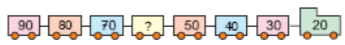2. Together, Chip and Dale have 8 nuts. They divide them equally. Chip ate 1 nut. How many nuts does he have left?

3. Calculate the sum of the numbers in the circle: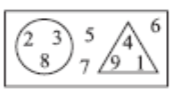4. Which digit does the flower cover up?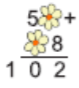5. In the class there are 20 students, 8 of whom are boys. At how many desks do the girls sit, if two of them sit at each desk?

6. How many meters does Ingo walk if he starts from A, goes through points B, C, D, and A?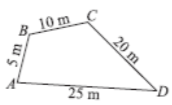7. A frog jumps on every rock, from rock number 3 to rock number 9. How many jumps does it make?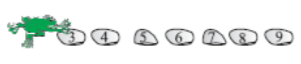8. After 1 comes 3, after 3 comes 5, after 5 comes 7. What comes after 7?
1  3  5  7 ...

9. 4 POINTS FOR EACH PROBLEM

Lucy, Maria and Anna have a meeting at 12:30. Lucy’s walk takes 10 minutes, Maria’s a quarter of an hour, and Anna’s 40 minutes. At what time must the person that needs the longest time to get to the meeting leave her house?

10. On each side of the triangle, the sum of the numbers is the same. A butterfly and a bee stopped to rest and they have covered up two numbers. On which number is the bee standing?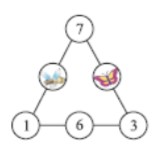11. How many bricks are missing in this wall?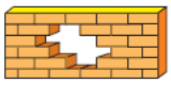12. Last year, the sum of ages of Iris, Beatrice and Lydia was 18 years. How old are they together this year?

13. How far does Cristi live from Andra?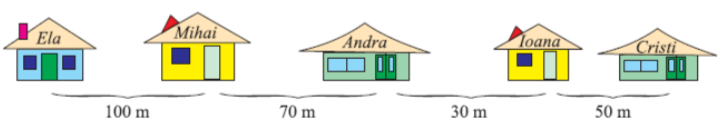14. Helen said to Mary, “If you give me 4 apples, I will have exactly as many apples as you.” How many more apples does Mary have than Helen?

15. On the farm, there are 5 sheep, 5 hens, 2 dogs, 2 cats and the farmer. How many legs are there altogether?

16. 5 POINTS FOR EACH PROBLEM

What is correct number in the question mark?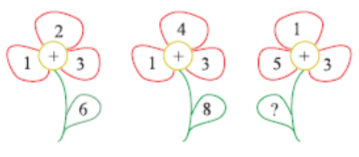17. Bibi the Turtle writes different numbers on flowers. She uses only the digits 1 and 2. On how many flowers can she write?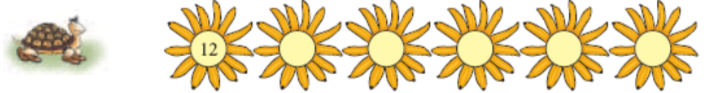18. Matthias received a book as a gift. He colored in it on pages 99 through 110. How many pages did Matthias color?

19. The first day of a certain month that has 30 days is a Monday. How many Mondays does this month have?

20. How many points did Andrew earn?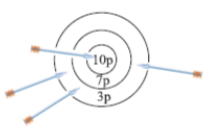21. Who is right?
Anna: “The largest number possible has 6 digits.”
Boris: “The largest number possible has 1000 digits.”
Caroline: “The largest number possible can be only written with the 9 digits.”
Daniel: “The largest number possible cannot be written.”
Else: “The largest number possible has 0 as the last digit.”

22. Which dots are covered?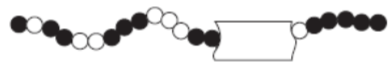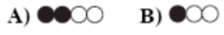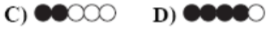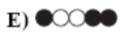23. As Hans wrote the problem on the board, he forgot one digit: 23 + 31 + 2 + 12 = 94. This digit is:

24. We place the signs +, – and = between the digits: 4 8 3 3 6 9 in such a way that an equation is built. The sequence of the signs is: